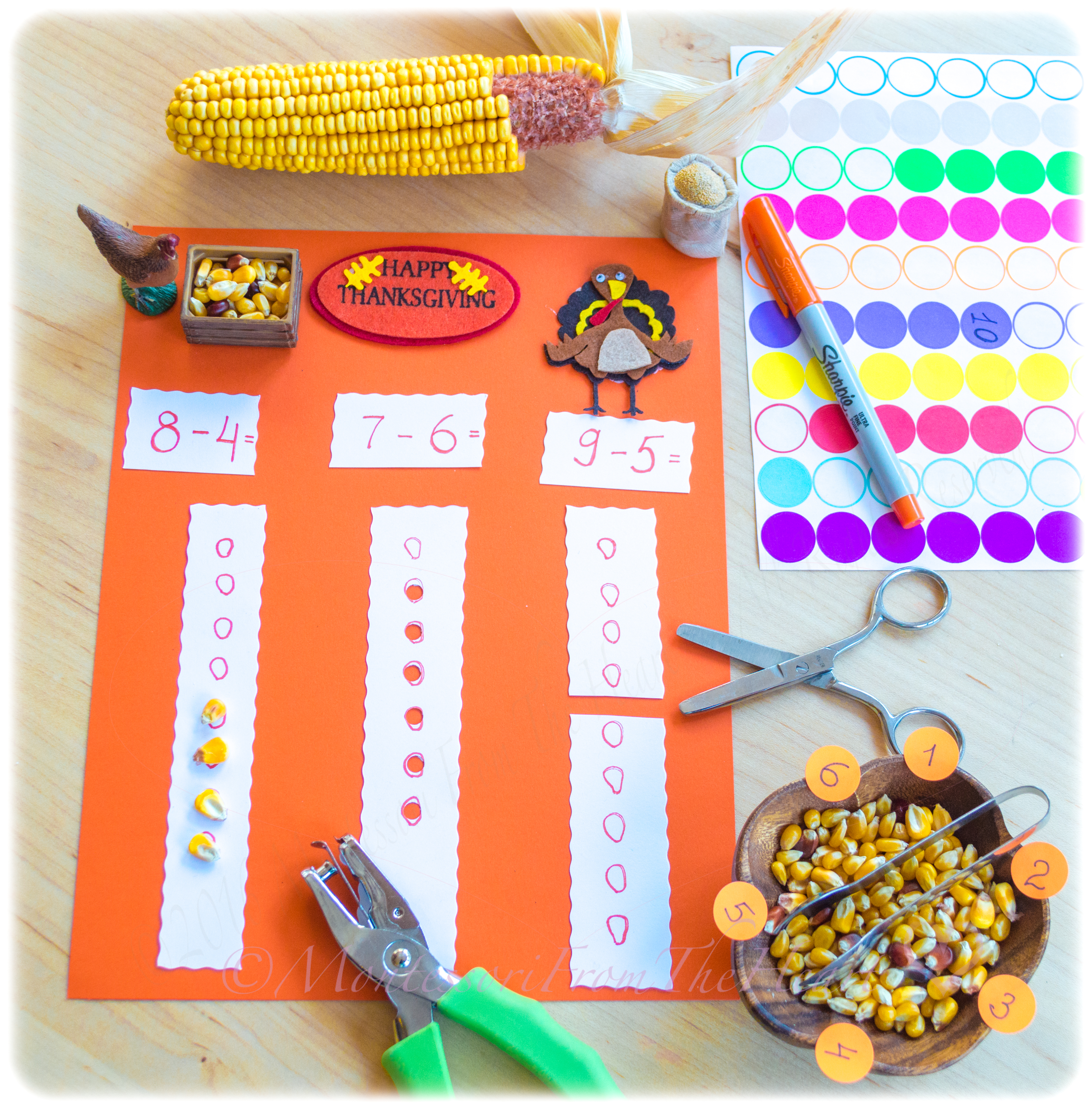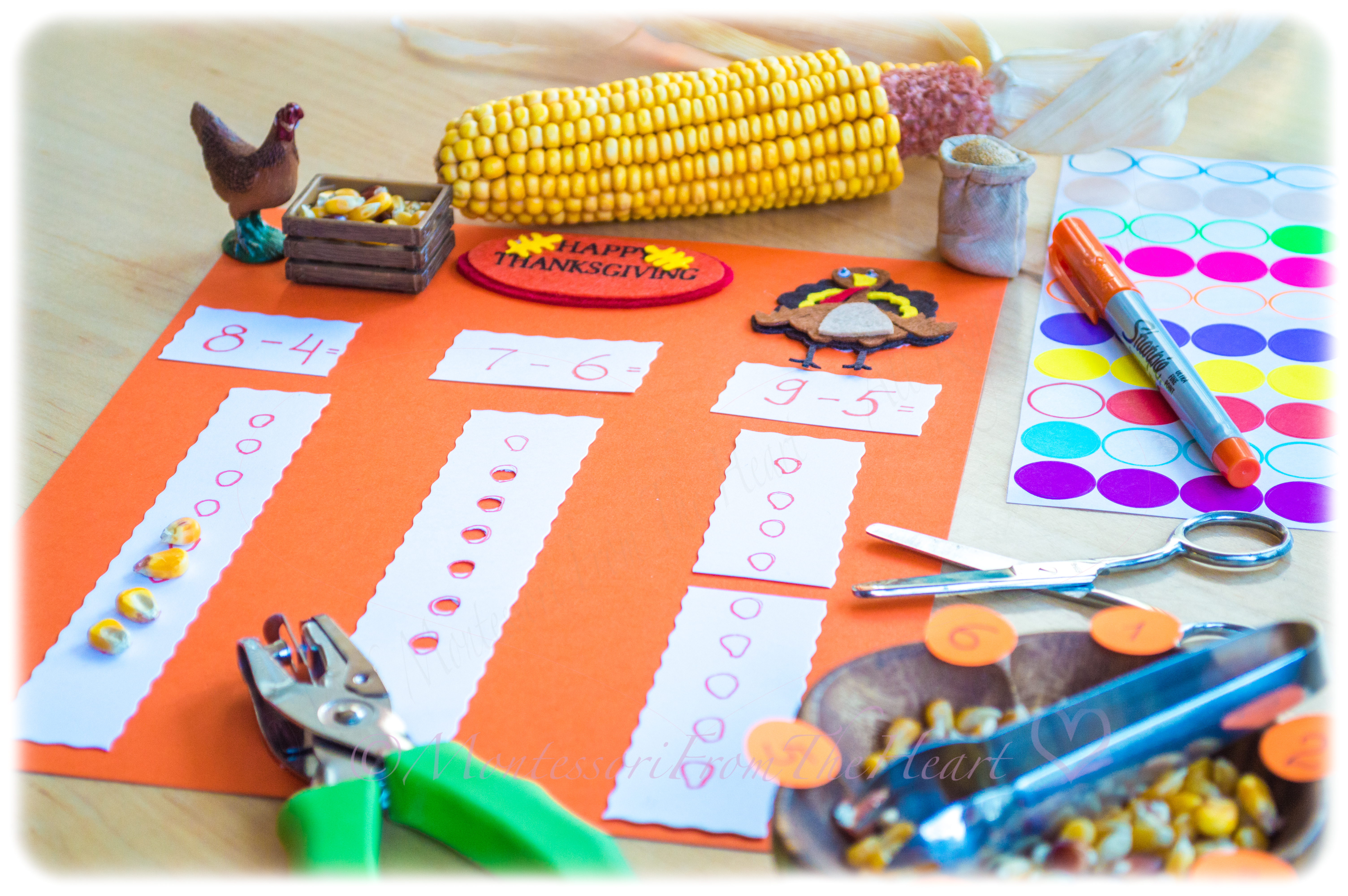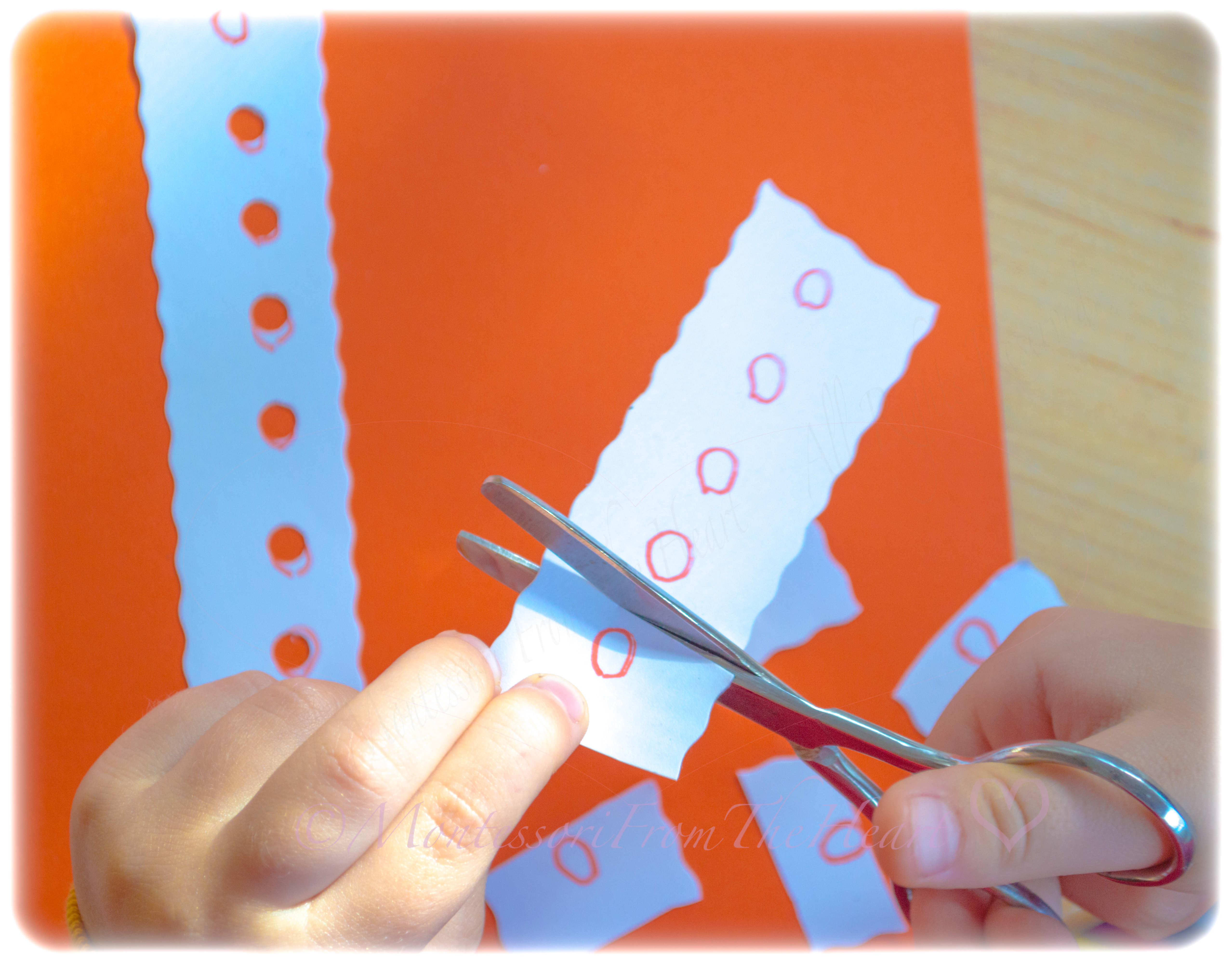This Thanksgiving Inspired DIY lesson on Subtraction uses materials you probably have at home. (Please note that this lesson should be presented to a child who already understands the concept of numerals at least till ten and the concept of addition; see the links below.)There are different ways you can explain the mathematical operation of "subtraction" to a child -  the process of taking away/removing objects from a collection: a 🦃 hen/turkey ate the kernels (thus the difference is less by what the turkey ate) or a child can use fine and gross motor control to punch out holes/kernels we are subtracting or to cut the subtrahend kernels.The 1st number (from which we are subtracting from) is called a minuend, while the number being subtracted (2nd number) is called the subtrahendThe result is called the difference.Subtracting/taking away can also be presented by cutting, thus resulting with less of something.Once the equation is solved, a child would choose the correct difference/result of the subtraction from number stickers.

See here a 🎥 video- post "Beginning ➖ Subtraction (Montessori 🔢 Math 🎥Lesson)." For Addition Math work, see here "Montessori Math Simple ➕ Addition using Marble-Counters" and here a 🎥 video-post "➕Addition Strip Board (Montessori 🔢 Math 🎥 Lesson)." Also see here an extension to Montessori Strip Board: "➕Addition Strip Board Making 🔟’s (Montessori 🔢 Math 🎥 Lesson)."

For more on themed unit studies, see here a summary post for the month of November "🦃Thanksgiving Inspired Homeschooling 101 Unit Study" and here a summary post with all activities we have done during the month of🍂September and🎃October in a post "🍂Fall & 🎃Halloween Inspired Homeschooling 101 Unit Study." Also here see what we did in 2016 "🦃Thanksgiving-inspired activities (2016). "